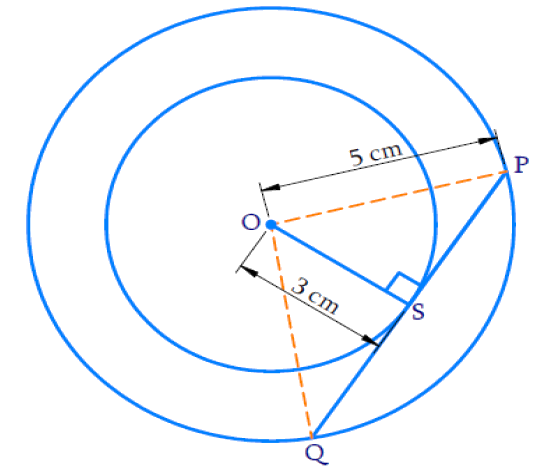# Ex.10.2 Q7 Circles Solution - NCERT Maths Class 10

Go back to  'Ex.10.2'

## Question

Two concentric circles are of radii $$\text{5 cm}$$ and $$\text{3 cm.}$$ Find the length of the chord of the larger circle which touches the smaller circle.

Video Solution
Circles
Ex 10.2 | Question 7

## Text Solution

What is Known?

Two concentric circles are of radii $$\text{5 cm}$$ and $$\text{3 cm.}$$

What is Unknown?

The length of the chord of the larger circle which touches the smaller circle.

Reasoning:

Chord of the larger circle is a tangent to the smaller circle.

Steps:$$PQ$$ is chord of a larger circle and tangent of a smaller circle. Tangent is perpendicular to the radius at the point of contact $$S.$$

$$\therefore \;\angle {OSP} = {90^ \circ }$$

In $$\Delta {OSP}$$ (Right angled triangle)

By the Pythagoras Theorem,

\begin{align} {O P} ^ { 2 } & = {O S} ^ { 2 } + {S P} ^ { 2 } \\ 5 ^ { 2 } & = 3 ^ { 2 } + {S P} ^ { 2 } \\ {S P} ^ { 2 } & = 25 - 9 \\ {S P} ^ { 2 } & = 16 \\ {S P} & = \pm 4 \end{align}

$$SP$$ is length of tangent and cannot be negative

$$\therefore \;SP = \rm{4\, cm}$$

$$QS = SP$$ (Perpendicular from center bisects the chord considering the larger circles)

Therefore, $$QS = SP = \text{4 cm}$$

Length of the chord

\begin{align} {PQ} & = \text{QS + SP} \\ & = 4 + 4 \\ { PQ} & = 8 \; \rm{ cm }\end{align}

Therefore, the length of the chord of the larger circle is $$\text{8 cm.}$$

Learn from the best math teachers and top your exams

• Live one on one classroom and doubt clearing
• Practice worksheets in and after class for conceptual clarity
• Personalized curriculum to keep up with school